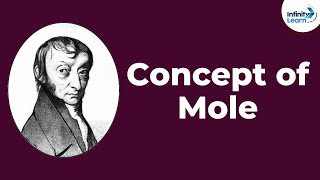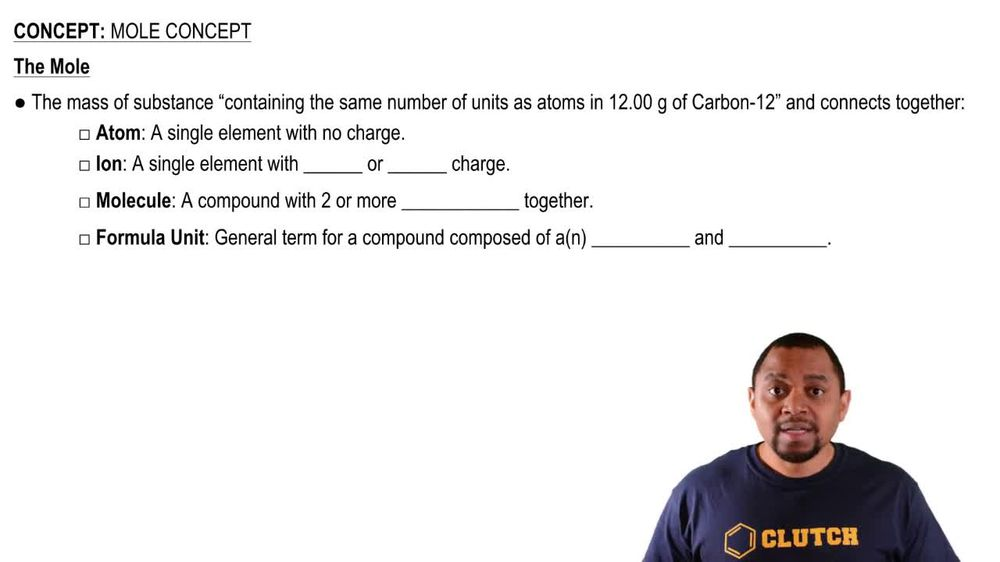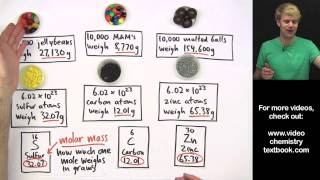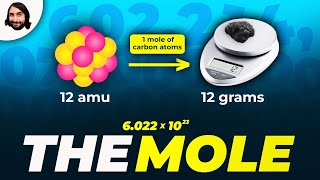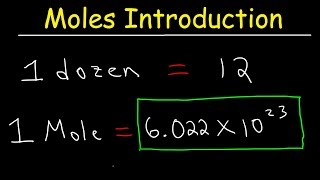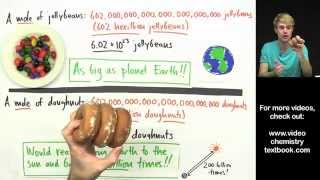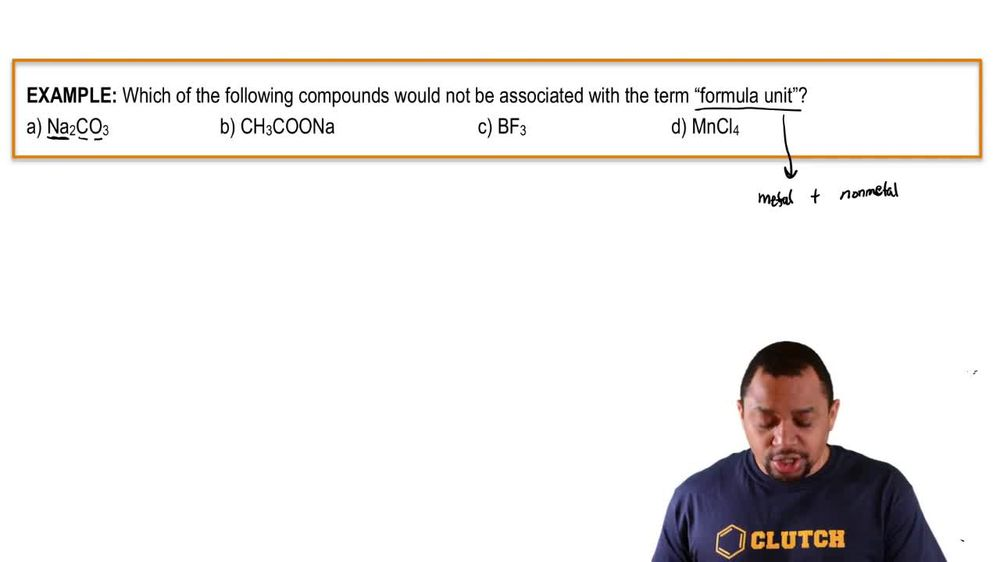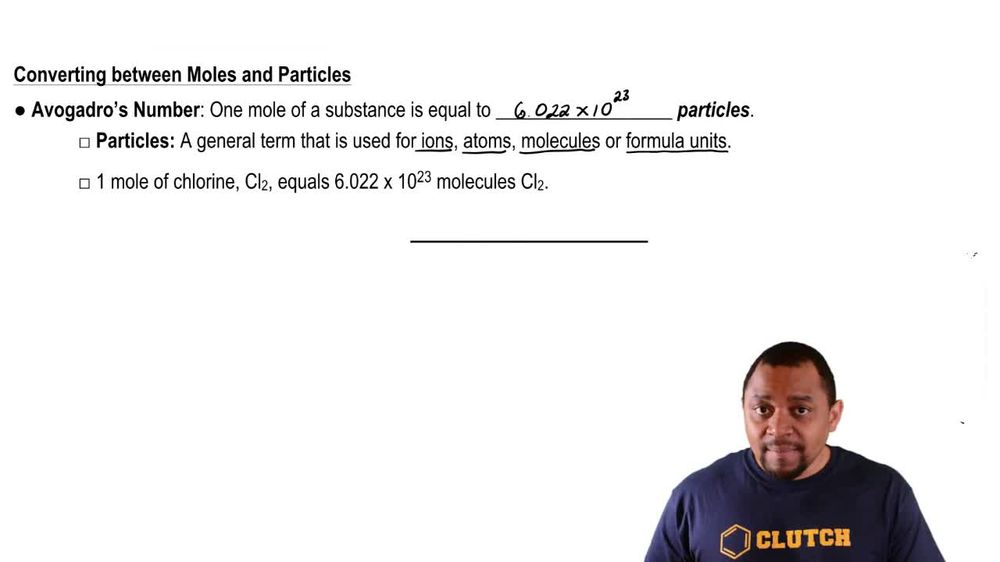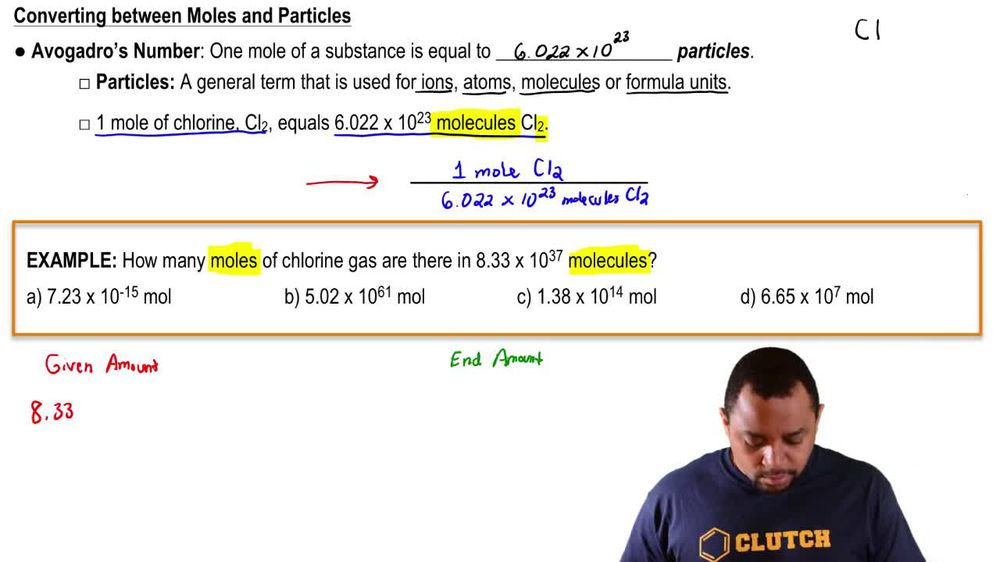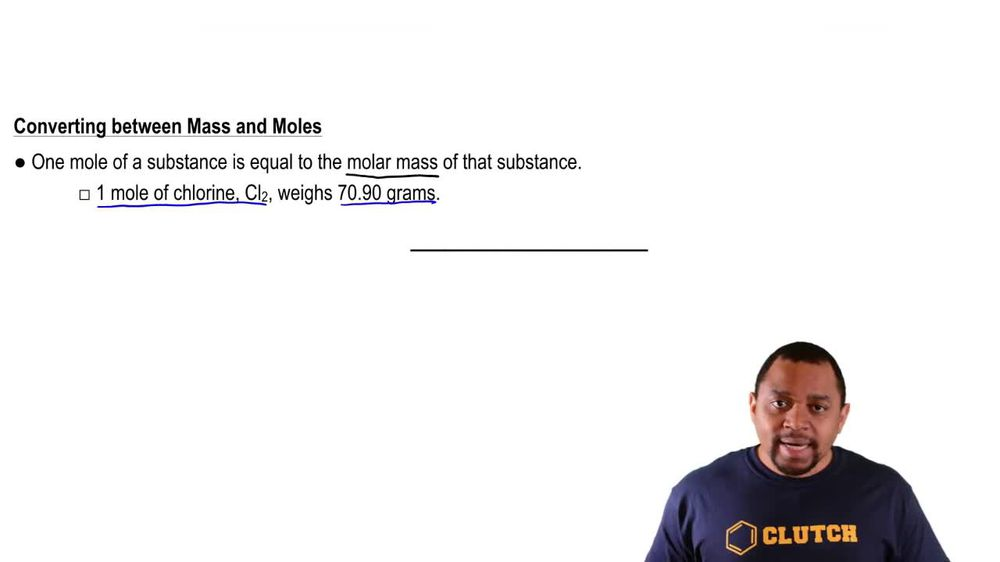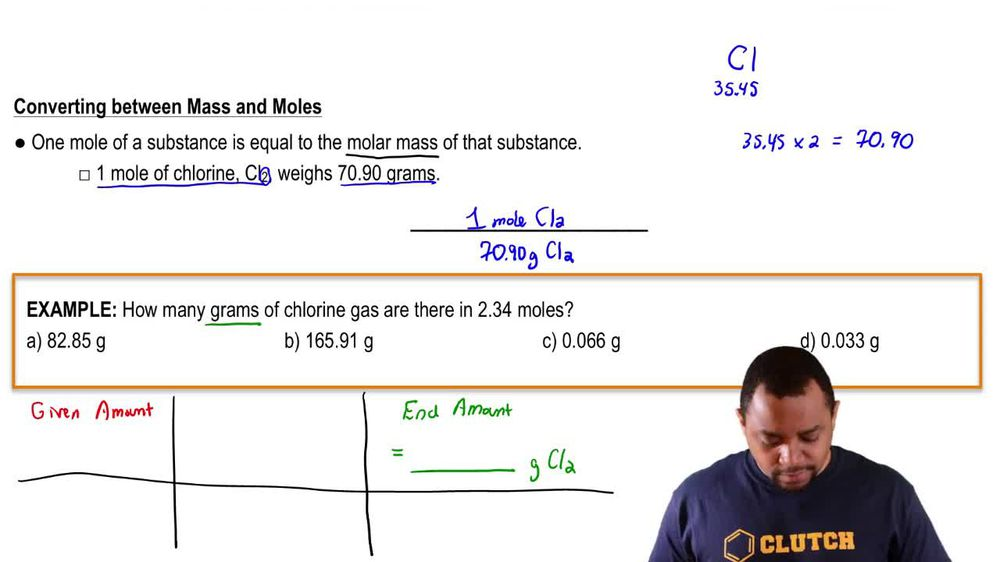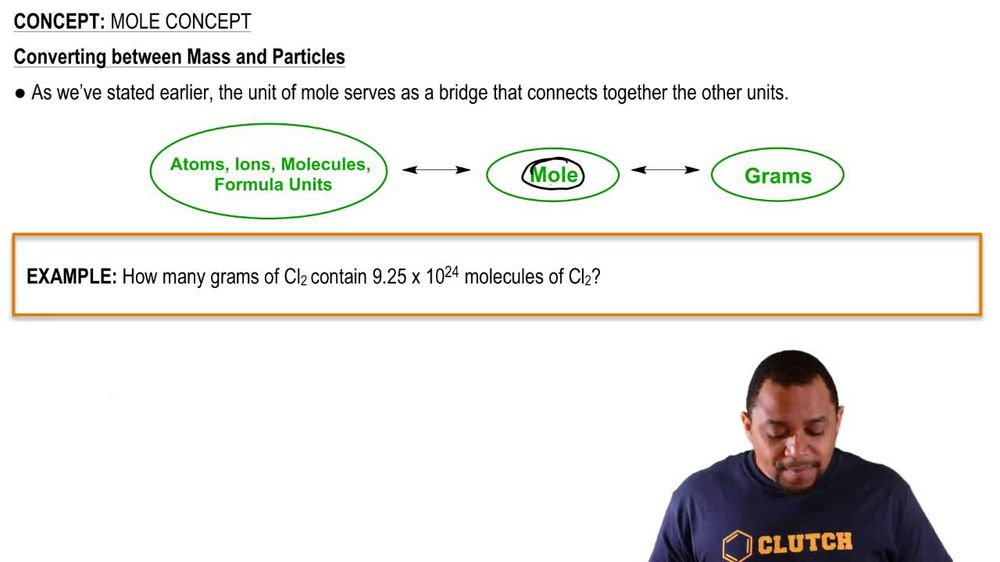Start typing, then use the up and down arrows to select an option from the list.
1. 2. Atoms & Elements2. Mole Concept
Problem

# The U.S. Environmental Protection Agency (EPA) sets limits on healthful levels of air pollutants. The maximum level that the EPA considers safe for lead air pollution is 1.5 mg>m3. If your lungs were filled with air containing this level of lead, how many lead atoms would be in your lungs? (Assume a total lung volume of 5.50 L.)

Relevant Solution2m
Play a video:
Hey everyone. Our question here states calculate the number of ethanol molecules in a cup with a volume of 236.6 ml with a density of 0.789 g per cubic centimeter first, Let's go ahead and determine the formula of ethanol. So we know that ethanol is going to be C two H five oh H. And we also need to recognize that one mil leader Is equivalent to one cc. So calculating the Mueller massive ethanol, We can see that we have two of carbon, which we're going to multiply by its atomic mass of 12.01g per mole, Which will get us to 24.02. Moving on to hydrogen, we have six of hydrogen and we're going to multiply that by its atomic mass of 1.008 Which will get us to a total of 6.048. And lastly looking at our oxygen, we have one of oxygen and we're going to multiply that by its atomic mass of 16. Now, when we add this all up, we end up with a molar mass of .068 g per mole. Now let's go ahead and answer our question. Starting off with our 236.6 ml we're going to convert the milliliters into cubic centimeters and now we're going to take our density of 0. grams per one cc. And now we're going to take the molar mass that we determined of 46.068 g of ethanol per one mole of ethanol. And in order to convert from moles into molecules, we know that one mole of ethanol is going to contain 6.02, 2 times 10 to the 23rd molecules of ethanol. And this is going to be avocados number. So once we calculate this out and cancel out our units, we're going to end up with a total of 2.4, 4 Times 10 to the ethanol molecules. And this is going to be our final answer. So I hope this made sense and let us know if you have any questions.